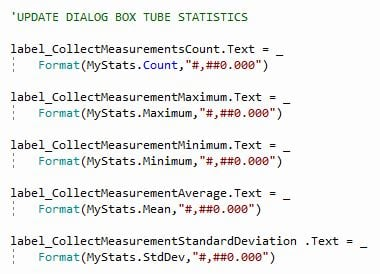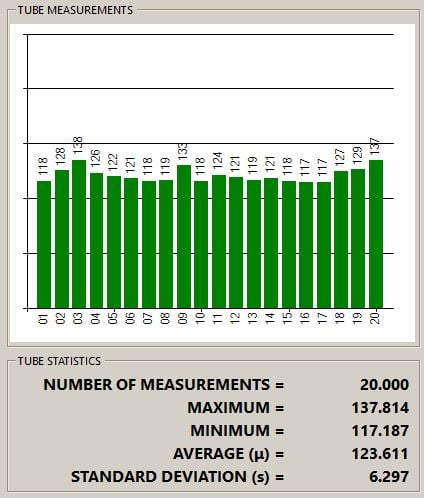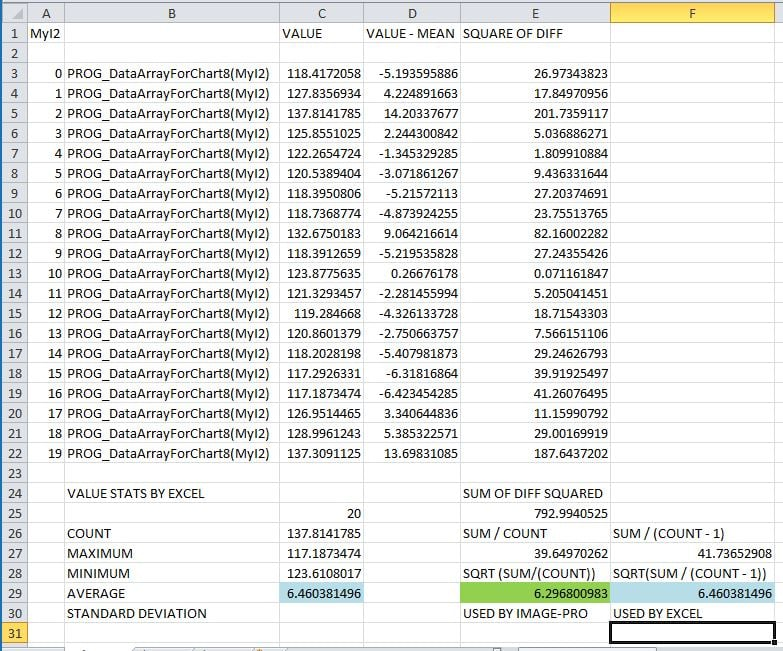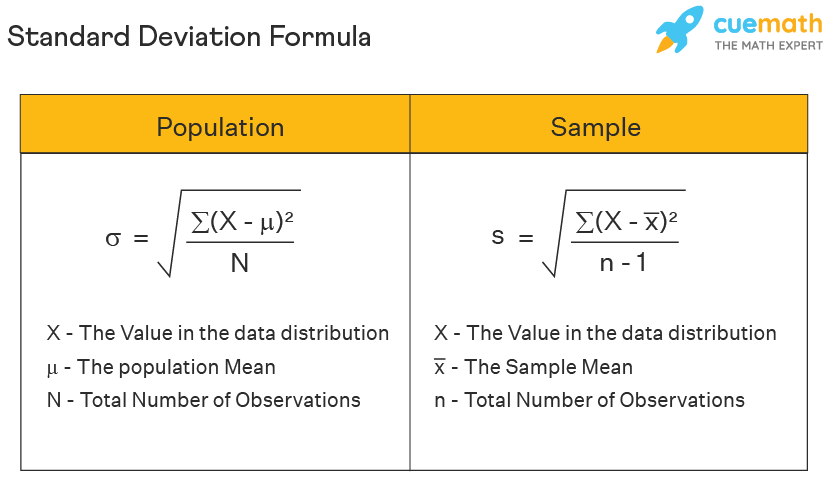# BEST CODE TO GET STATISTICS FOR AN ARRAY OR A LIST . . .

2023-01-16-155334

All --

I am working on an APP that has a bunch of different ARRAYS of MEASUREMENTS from a bunch of different IMAGES.

The APP needs to be able to CALCULATE and DISPLAY the STATISTICS for each of the ARRAYS.

In a DISCUSSION in MAY-2015, I was directed to

ThisApplication.GlobalTools.McBasicStatistics

and that works but at some point I was able to use LISTS and CODE for VB.NET that gets the STATISTICS with something like

ListName.StdDev

This was discussed in a DISCUSSION in MAR-2021 but I cannot seem to get the LISTS to show the STATISTICAL TOOLS like I seemed to be able to when I did this

***

After declaring a LIST in IP10 with something like this

`    Dim Prog_ListLengths1 As New System.Collections.Generic.List(Of Double)`

and then adding my Lengths to the LIST with something like this

```                                    'REMEMBER THE LENGTH OF THIS FEATURE
( _
sf.Value(eMeasures.RgnLength) _
)```

Then I can get the STATS from the LIST with something like this

```                Debug.Print Prog_ListLengths1.Min
Debug.Print Prog_ListLengths1.Max
Debug.Print Prog_ListLengths1.Average```

***

I have created and attached a DEMONSTRATION PROJECT in

Array Statistics Project V1A.ipx

that has a DEMO ARRAY, a DEMO LIST, and a determination of a STANDARD DEVIATION using

```        Dim MyStats As mediacy.IQL.ObjectManager.BASIC_STATISTICS = _
ThisApplication.GlobalTools.McBasicStatistics(MyDemoArray)

textBox3.Text = _
"The STANDARD DEVIATION for MyDemoArray is " & Format (MyStats.StdDev,"#,##0.00")```

Is there a better way to get the STATISTICS for the DEMO ARRAY or the DEMO LIST?

Thanks.

-- Matt

• edited January 17 Answer ✓
Hi Matt,

System.Linq with .NET IEnumerable interface provides functions for simple statistics, such as Min, Max, Sum, Average. Check this link: https://learn.microsoft.com/en-us/dotnet/api/system.linq.enumerable?view=netframework-4.8

If you need Standard Deviation for any array, then you have to use something else, such as McBasicStatistics.

If you are trying to get statistics of Measurements, then you can use Statistics property of McMMData: https://help.mediacy.com/Image-Pro/Automation/11/html/Properties_T_MediaCy_Addins_Measurements_StatsClass.htm

Here is an example that prints standard deviation of Area of the objects in active image:

```    Public Sub MeasurementStats()
Debug.Print (\$"Standard deviation of Area = {md.Statistics(MediaCy.Addins.Measurements.eMeasures.RgnArea).StdDev}")
End Sub
```

Yuri

• Hi Matt,

As you pointed out, there are different ways to calculate Standard Deviation. Wikipedia page shows 3 equations (uncorrected, corrected, unbiased) : https://en.wikipedia.org/wiki/Standard_deviation

Image-Pro uses the "uncorrected" formula with N in denominator (also called standard deviation of entire population).  Excel also has this function, it's called STDEVPA. The difference between STDEVA and STDEVPA is very small for large number of objects.

If you need a specific version, you can use the conversion formula as in your sample.

Yuri

• Yuri —

Thanks for that info.

These arrays are filled with one measurement per image and grouped by sample preparation group.

I think MCBASICSTATISTICS is the way to go.

Having the STATISTICS available for MEASUREMENTS from a COUNT in the manner you reviewed is very help and I have used it previously.

I thought that there was a way to declare a LIST in a manner that extensions like .STDDEV retuned STATISTICS for the LIST.

Thanks again.

— Matt
• 2023-01-17-180909

Yuri --

I have used

MCBASICSTATISTICS

to calculate the STATISTICS for an ARRAY.

I have passed those STATISTICS back to the DIALOG BOX using this codeThe values in the ARRAY and shown in the LABELS is shown hereWhen I transfer the VALUES in the ARRAY to EXCEL and calculate the STATISTICS, the results are shown here.The STANDARD DEV calculated by IMAGE-PRO is 6.29.  The STANDARD DEVIATION calculated by EXCEL is 6.46.

The difference seems to be whether the STANDARD DEVIATION is calculated using the denominator or N or N - 1.

EXCEL seems to use the N - 1 method labeled POPULATION in the image below.

IMAGE-PRO seems to use the N method labeled SAMPLE in the image below.Most references that I see use the use the N - 1 method.

I can get the same value as the N method by using the IMAGE-PRO STATISTICS

label_CollectMeasurementStandardDeviation .Text = _
Format(System.Math.Sqrt((MyStats.Variance)*(MyStats.Count)/(MyStats.Count-1)),"#,##0.000")

Is there is a reason that IMAGE-PRO varies from EXCEL on this?

Thanks.

-- Matt